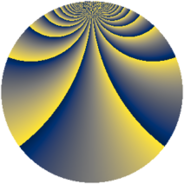# Properties

 Label 1148.2.cgLevel $1148$ Weight $2$ Character orbit 1148.cg Rep. character $\chi_{1148}(87,\cdot)$ Character field $\Q(\zeta_{60})$ Dimension $2624$ Sturm bound $336$

# Learn more about

## Defining parameters

 Level: $$N$$ $$=$$ $$1148 = 2^{2} \cdot 7 \cdot 41$$ Weight: $$k$$ $$=$$ $$2$$ Character orbit: $$[\chi]$$ $$=$$ 1148.cg (of order $$60$$ and degree $$16$$) Character conductor: $$\operatorname{cond}(\chi)$$ $$=$$ $$1148$$ Character field: $$\Q(\zeta_{60})$$ Sturm bound: $$336$$

## Dimensions

The following table gives the dimensions of various subspaces of $$M_{2}(1148, [\chi])$$.

Total New Old
Modular forms 2752 2752 0
Cusp forms 2624 2624 0
Eisenstein series 128 128 0

## Trace form

 $$2624q - 10q^{2} - 6q^{4} - 60q^{5} - 40q^{8} + O(q^{10})$$ $$2624q - 10q^{2} - 6q^{4} - 60q^{5} - 40q^{8} - 18q^{10} - 24q^{12} - 26q^{14} - 6q^{16} - 48q^{17} - 30q^{18} - 40q^{21} - 64q^{22} + 30q^{24} + 284q^{25} - 42q^{26} - 54q^{28} - 128q^{29} - 26q^{30} - 60q^{33} - 120q^{36} - 12q^{37} - 66q^{38} - 108q^{40} - 64q^{42} + 8q^{44} - 36q^{45} - 110q^{46} - 40q^{49} - 84q^{52} - 16q^{53} - 96q^{54} - 102q^{56} - 48q^{57} + 2q^{58} - 30q^{60} - 60q^{61} + 12q^{65} + 162q^{66} - 210q^{68} - 70q^{70} + 130q^{72} - 10q^{74} - 40q^{77} - 16q^{78} - 30q^{80} + 944q^{81} - 300q^{82} - 20q^{84} - 136q^{85} + 2q^{86} - 38q^{88} + 72q^{89} + 172q^{92} - 56q^{93} - 6q^{94} - 180q^{96} + 82q^{98} + O(q^{100})$$

## Decomposition of $$S_{2}^{\mathrm{new}}(1148, [\chi])$$ into newform subspaces

The newforms in this space have not yet been added to the LMFDB.